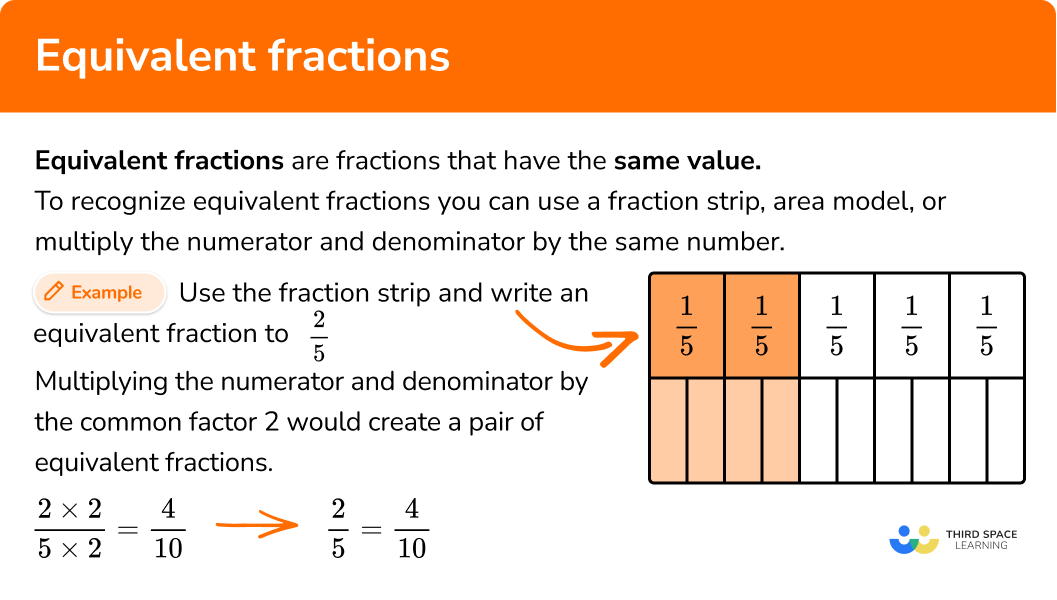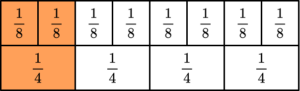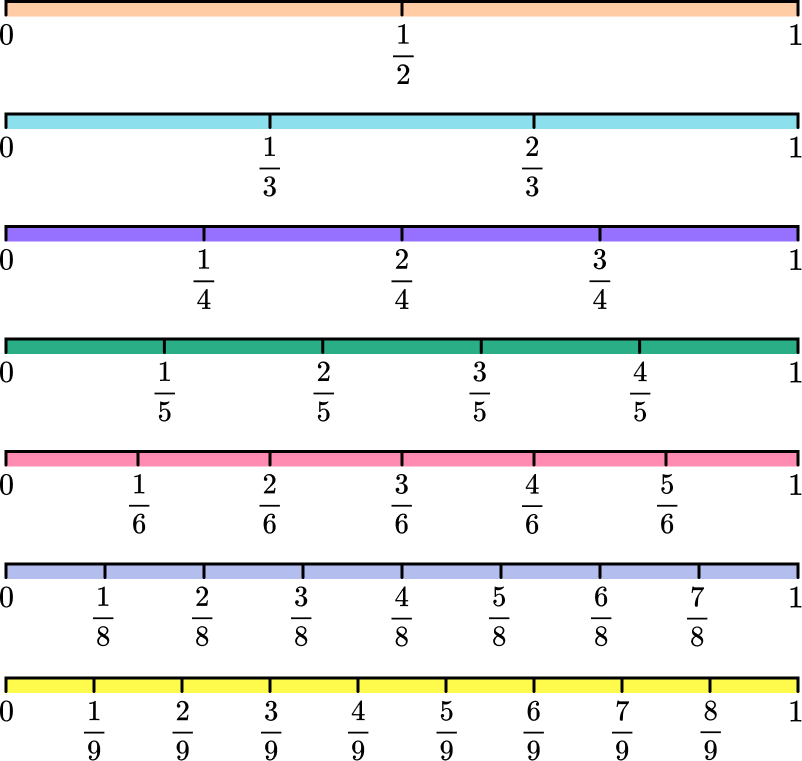# Equivalent fractions

Here you will learn about equivalent fractions including how to simplify fractions and how to find equivalent fractions.

Students will first learn about equivalent fractions as part of number and operations in fractions in elementary school.

## What are equivalent fractions?

Equivalent fractions are fractions that have the same value. They help us to compare fractions, list them in order from least to greatest and write in lowest terms.

• You can use area models to find equivalent fractions.

For example, find an equivalent fraction to \cfrac{1}{2}

\; \cfrac{1\times2}{2\times2}=\cfrac{2}{4} \hspace{1cm} \cfrac{1\times4}{2\times4}=\cfrac{4}{8} \hspace{1cm} \cfrac{1\times3}{2\times3}=\cfrac{3}{6}

For example, find an equivalent fraction to \cfrac{2}{3}

\; \cfrac{2\times3}{3\times3}=\cfrac{6}{9}

Notice that the numerator and the denominator were both multiplied by the same common factor of 3.

• You can also use fraction strips to find equivalent fractions.

For example, find an equivalent fraction to \cfrac{2}{5}

Notice that the numerator and denominator were multiplied by a common factor of 2.

\cfrac{2\times2}{5\times2}=\cfrac{4}{10}

\cfrac{2}{5}=\cfrac{4}{10}

For example, find an equivalent fraction to \cfrac{5}{6}

Notice that the numerator and denominator were multiplied by the whole number 2.

\cfrac{5 \times 2}{6 \times 2}=\cfrac{10}{12}

\cfrac{5}{6}=\cfrac{10}{12}

### What are equivalent fractions?## Common Core State Standards

How does this relate to 4th grade math and 5th grade math?

• Grade 3 – Numbers & Operations – Fractions (3.NF.A.3.b)
Recognize and generate simple equivalent fractions, (e.g., \cfrac{1}{2}=\cfrac{2}{4}, \; \cfrac{4}{6}=\cfrac{2}{3} ). Explain why the fractions are equivalent, e.g., by using a visual fraction model.
• Grade 4 – Numbers & Operations – Fractions (4.NF.A.1)
Explain why a fraction \cfrac{a}{b} is equivalent to a fraction \cfrac{(n \times a)}{(n \times b)} by using visual fraction models, with attention to how the number and size of the parts differ even though the two fractions themselves are the same size. Use this principle to recognize and generate equivalent fractions.

## How to write equivalent fractions

In order determine if the given fractions are equivalent:

1. Compare the numerators and denominators of the given fractions.
2. Using either multiplication or division, find the common factor that was multiplied or divided from the first fraction to the second fraction.
3. Write the equivalent fractions.

In order to find a missing denominator or numerator of equivalent fractions:

1. Find the common factor.
2. Multiply or divide the numerator and denominator of one of the fractions by the common factor.
3. Write the equivalent fraction.

## Equivalent fractions examples

### Example 1: fraction strip to determine if fractions are equivalent

Determine if the fractions represented by the fraction strip are equivalent.

1. Compare the numerators and denominators of the given fractions.

The denominator of the first fraction is 4 with 3 equal shaded parts. \cfrac{3}{4}

The denominator of the second fraction is 8 with 6 equal shaded parts. \cfrac{6}{8}

2Using either multiplication or division, find the common factor that was multiplied or divided from the first fraction to the second fraction.

Using multiplication, the common factor is 2.

\cfrac{3\times 2}{4\times 2}=\cfrac{6}{8}

3Write the equivalent fractions.

\cfrac{3}{4}=\cfrac{6}{8}

### Example 2: area model to determine if fractions are equivalent

Determine if the fractions represented by the area models are equivalent.

The first circle is divided into 16 equal parts with 6 parts shaded. \cfrac{6}{16}

The second circle is divided into 8 equal parts with 3 parts shaded. \cfrac{3}{8}

Using division, the common factor is 2.

\cfrac{6\div 2}{16\div 2}=\cfrac{3}{8}

\cfrac{6}{16}=\cfrac{3}{8}

### Example 3: find the equivalent fraction

Which fraction is equivalent to \cfrac{12}{18}?

\cfrac{4}{7}, \; \cfrac{2}{3}, \; \cfrac{1}{6}

\cfrac{12}{18} \cfrac{4}{7} \; The numerators and denominators do NOT have the same common factor.

\cfrac{12}{18}=\cfrac{2}{3} \; The numerators and denominators do have the same common factor. ✔

\cfrac{12}{18} \cfrac{1}{6} \; The numerators and denominators do NOT have the same common factor.

\cfrac{12\div 6}{18\div 6}=\cfrac{2}{3} \; The common factor is 6. Divide the numerator and denominator by 6.

\cfrac{12}{18}=\cfrac{2}{3}

### Example 4: find the missing denominator

Find the missing denominator.

\cfrac{3}{5}=\cfrac{15}{?}

The numerator for the first fraction is 3 and the numerator of the second fraction is 15. The first numerator was multiplied by a common factor of 5 to get the second numerator of 15.

\cfrac{3\times 5}{5\times ?}=\cfrac{15}{?}

Multiply the numerator and denominator by the common factor 5.

\cfrac{3\times5}{5\times5}=\cfrac{15}{?}

\cfrac{15}{25}

### Example 5: find the missing numerator

Find the missing numerator.

\cfrac{?}{20}=\cfrac{3}{5}

\cfrac{?}{20}=\cfrac{3}{5}

The denominators of the given fractions are 20 and 5.

20 \div 5 = 4

So the common factor is 4.

\cfrac{?}{20}=\cfrac{3\times 4}{5\times 4}

\cfrac{12}{20}

### Example 6: find the missing numerator.

Find the missing numerator.

\cfrac{24}{63}=\cfrac{?}{21}

\cfrac{24}{63}=\cfrac{?}{21}

The denominators of the given fractions are 63 and 21.

63 \div 3 = 21

So the common factor is 3.

\cfrac{24\div 3}{63\div 3}=\cfrac{?}{21}

\cfrac{24}{63}=\cfrac{8}{21}

### Teaching tips for equivalent fraction

• Instead of giving students a fraction worksheet to practice, consider having students actively engage with concrete models such as fraction strips or area models to help students visualize and formulate understanding of equivalent fractions.

• Number lines are a powerful visual tool for teaching equivalent fractions because it helps students to understand the correlation between fractions with the same and different denominators as well as the same and different numerators.

• Completely simplified fractions are the same as fractions in lowest terms.

• The cross multiplication strategy for equivalent fractions is not the most effective strategy. It will confuse students as they move into ratios and proportions.

### Easy mistakes to make

• Students may add or subtract the same number to form equivalent fractions instead of multiply or divide
You cannot make equivalent fractions by using addition.
For example, this is an incorrect simplifying technique.
\cfrac{5}{10}=\cfrac{2+3}{7+3}=\cfrac{2}{7}
This is the correct simplifying technique.
\cfrac{5}{10}=\cfrac{1\times5}{2\times5}=\cfrac{1}{2}

• Thinking fractions with different numerators and denominators can’t be equivalent
For example, \cfrac{5}{10} and \cfrac{1}{2}
Because the two given fractions do not have the same numerical numerator and denominator, they can be mistaken as not equal. Using common factor strategies or visual models help students formulate understanding.

• Multiplying or dividing the numerator and denominator by different numbers
For example, taking \cfrac{2}{3} and multiplying the numerator by 3 and the denominator by 4.
\cfrac{2\times 3}{3\times 4}=\cfrac{6}{12}
\cfrac{2}{3} \cfrac{6}{12}

• Thinking the denominator and the numerator are the same

### Practice equivalent fractions questions

1.Using the area models, represent the shaded area as equivalent fractions.

\cfrac{3}{6}=\cfrac{6}{18}\cfrac{2}{5}=\cfrac{6}{15}\cfrac{3}{5}=\cfrac{9}{15}\cfrac{2}{5}=\cfrac{5}{8}The first circle is divided into 5 equal parts with 3 parts shaded. \cfrac{3}{5}

The second circle is divided into 15 equal parts with 9 parts shaded. \cfrac{9}{15}

The equivalent fractions are \cfrac{3}{5}=\cfrac{9}{15}.

2. Which equivalent statement is true?\cfrac{1}{8}=\cfrac{1}{4}\cfrac{2}{8}=\cfrac{2}{4}\cfrac{2}{8}=\cfrac{1}{4}\cfrac{1}{4}=\cfrac{3}{8}Looking at the fraction strip the top row has two shaded parts which equals \cfrac{2}{8} and the second row has one shaded part which equals \cfrac{1}{4}.

The equivalent fractions are \cfrac{2}{8}=\cfrac{1}{4}.

The common factor is 2.

\cfrac{2\div 2}{8\div 2}=\cfrac{1}{4}

3. Which fraction is equivalent to \cfrac{63}{108}?

\cfrac{9}{12}\cfrac{7}{12}\cfrac{7}{9}\cfrac{3}{9}Compare the numerator and denominator of the given fraction to the numerators and denominators of the answer choices to find a common factor. The common factor is 9.

\cfrac{63\div 9}{108\div 9}=\cfrac{7}{12}

\cfrac{63}{108}=\cfrac{7}{12}

4. Find the missing numerator.

\cfrac{3}{4}=\cfrac{?}{24}

\cfrac{21}{24}\cfrac{23}{24}\cfrac{19}{24}\cfrac{18}{24}\cfrac{3}{4}=\cfrac{3\times 6}{4\times 6}=\cfrac{18}{24}

18 is the missing numerator.

5. Find the missing denominator.

\cfrac{6}{7}=\cfrac{24}{?}

\cfrac{24}{28}\cfrac{24}{26}\cfrac{24}{25}\cfrac{24}{27}\cfrac{6}{7}=\cfrac{6\times 4}{7\times 4}=\cfrac{24}{28}

28 is the missing denominator.

6. Find the missing denominator.

\cfrac{24}{?}=\cfrac{3}{13}

10483972\cfrac{3}{13}=\cfrac{3\times 8}{13\times 8}=\cfrac{24}{104}

104 is the missing denominator.

## Equivalent fractions FAQs

Why are equivalent fractions important?

Equivalent fractions and “fraction families” are used to help us add and subtract fractions with unlike denominators and find common denominators. Equivalent fractions also help us to write fractions in simplest form.

Are there other strategies to find equivalent fractions?

Yes, using number lines is a powerful tool with fractions. It helps build understanding when comparing fractions with different denominators, different numerators, and building number sense.## Still stuck?

At Third Space Learning, we specialize in helping teachers and school leaders to provide personalized math support for more of their students through high-quality, online one-on-one math tutoring delivered by subject experts.

Each week, our tutors support thousands of students who are at risk of not meeting their grade-level expectations, and help accelerate their progress and boost their confidence.